Publicité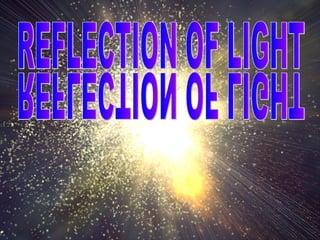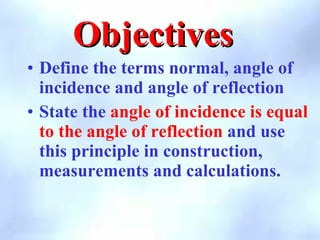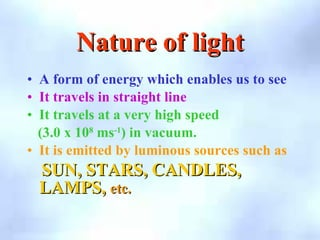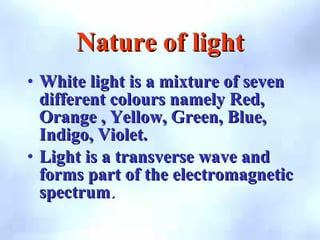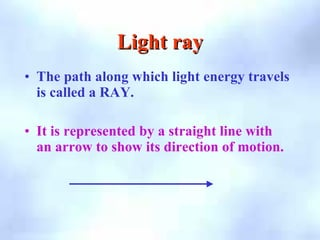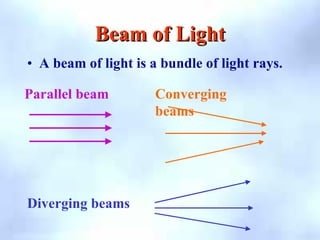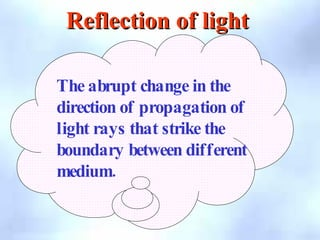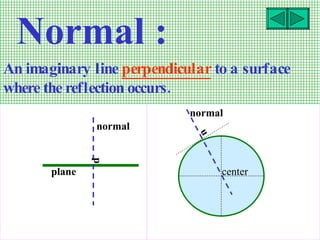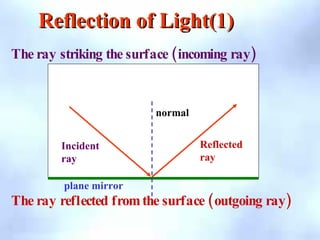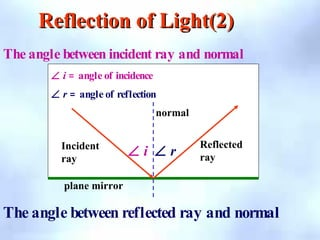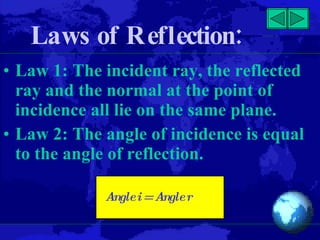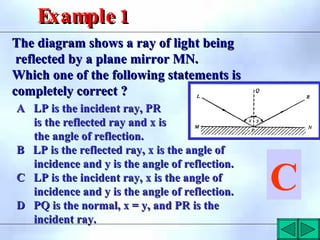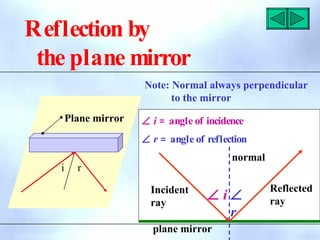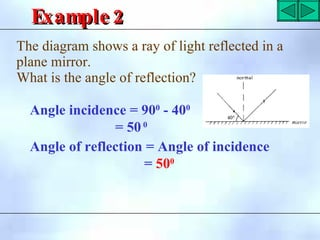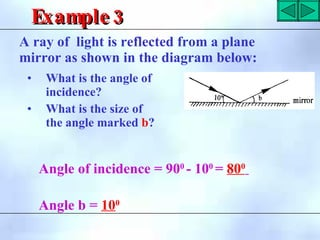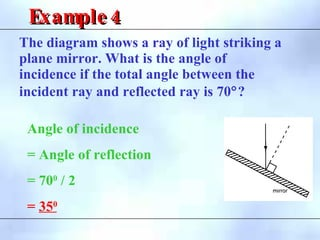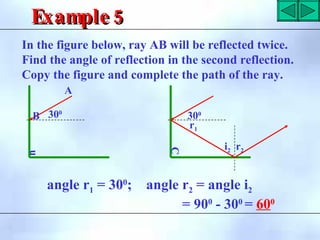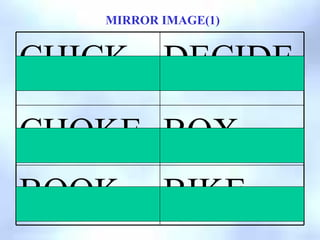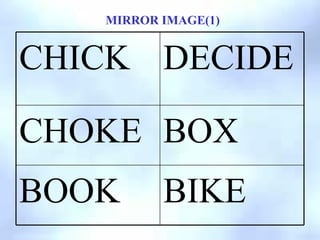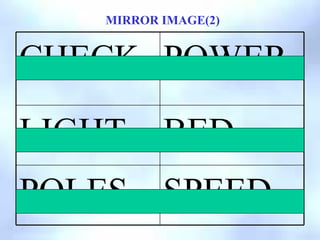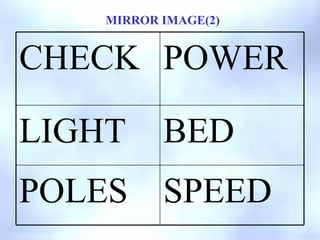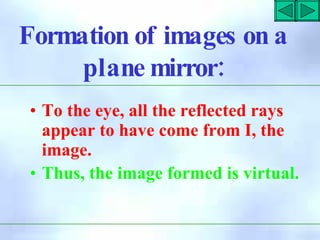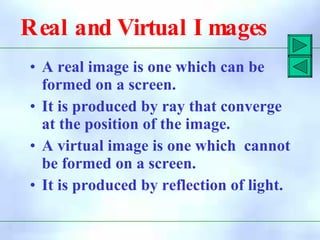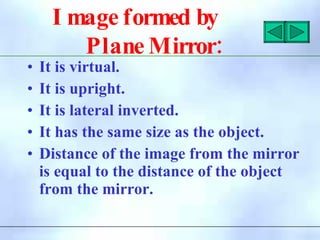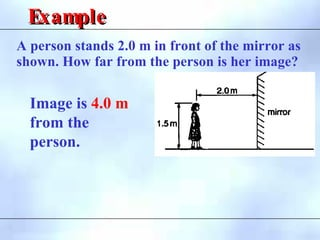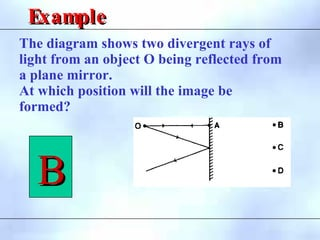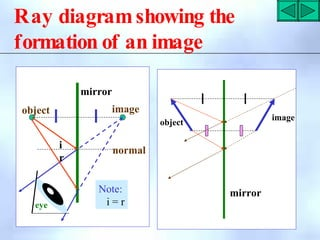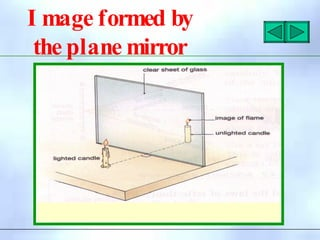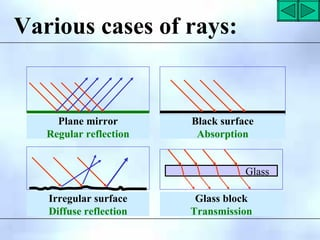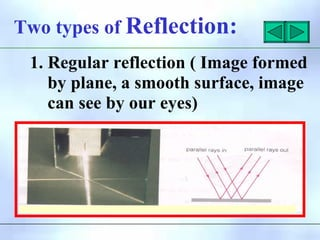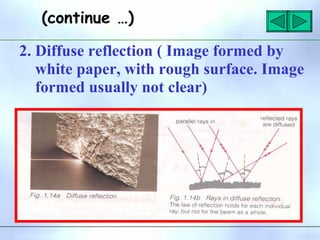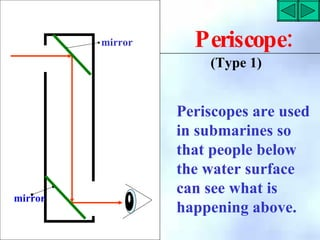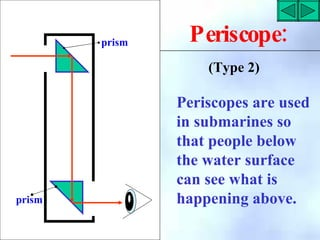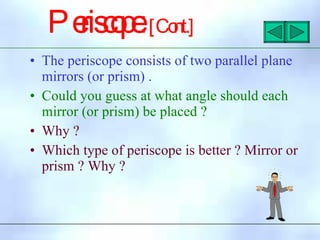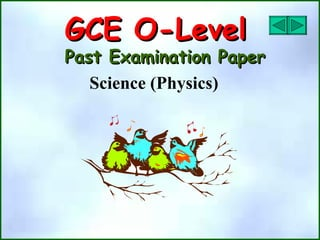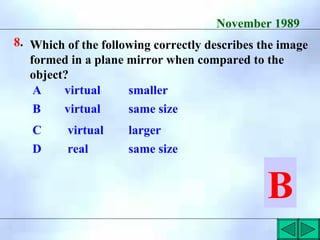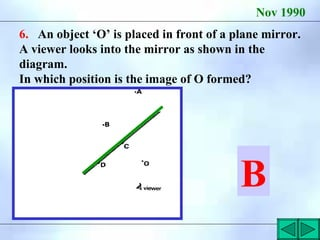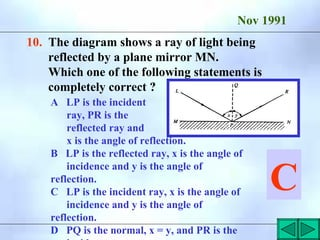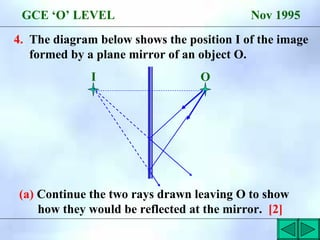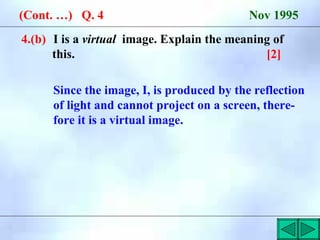1 sur 40
Publicité

### reflectionoflight-100829070425-phpapp02 (1).pdf

1. Objectives Objectives • Define the terms normal, angle of incidence and angle of reflection • State the angle of incidence is equal to the angle of reflection and use this principle in construction, measurements and calculations.
2. Nature of light Nature of light • A form of energy which enables us to see • It travels in straight line • It travels at a very high speed (3.0 x 108 ms-1 ) in vacuum. • It is emitted by luminous sources such as SUN, STARS, CANDLES, SUN, STARS, CANDLES, LAMPS, LAMPS, etc. etc.
3. Nature of light Nature of light • White light is a mixture of seven White light is a mixture of seven different colours namely Red, different colours namely Red, Orange , Yellow, Green, Blue, Orange , Yellow, Green, Blue, Indigo, Violet. Indigo, Violet. • Light is a transverse wave and Light is a transverse wave and forms part of the electromagnetic forms part of the electromagnetic spectrum spectrum. .
4. Light ray Light ray • The path along which light energy travels is called a RAY. • It is represented by a straight line with an arrow to show its direction of motion.
5. Beam of Light Beam of Light • A beam of light is a bundle of light rays. Parallel beam Converging beams Diverging beams
6. The abrupt change in the direction of propagation of light rays that strike the boundary between different . medium Reflection of light Reflection of light
7. plane p normal u normal center An imaginary line perpendicular to a surface . where the reflection occurs Normal :
8. Reflection of Light(1) Reflection of Light(1) i r Incident ray normal plane mirror ( ) The ray striking the surface incoming ray ( ) The ray reflected fromthe surface outgoing ray Reflected ray
9. Reflection of Light(2) Reflection of Light(2) The angle between incident ray and normal The angle between reflected ray and normal i r Incident ray normal plane mirror Reflected ray ∠ i = angle of incidence ∠ r = angle of reflection ∠ i ∠ r
10. : Laws of Reflection • Law 1: The incident ray, the reflected ray and the normal at the point of incidence all lie on the same plane. • Law 2: The angle of incidence is equal to the angle of reflection. Anglei=Angler
11. The diagram shows a ray of light being The diagram shows a ray of light being reflected by a plane mirror MN. reflected by a plane mirror MN. Which one of the following statements is Which one of the following statements is completely correct ? completely correct ? A LP is the incident ray, PR A LP is the incident ray, PR is the reflected ray and x is is the reflected ray and x is the angle of reflection. the angle of reflection. B LP is the reflected ray, x is the angle of B LP is the reflected ray, x is the angle of incidence and y is the angle of reflection. incidence and y is the angle of reflection. C LP is the incident ray, x is the angle of C LP is the incident ray, x is the angle of incidence and y is the angle of reflection. incidence and y is the angle of reflection. D PQ is the normal, x = y, and PR is the D PQ is the normal, x = y, and PR is the incident ray. incident ray. C 1 Example1 Example
12. Reflection by the plane mirror i r Plane mirror Note: Normal always perpendicular to the mirror i r Incident ray normal plane mirror Reflected ray ∠ i = angle of incidence ∠ r = angle of reflection ∠ i ∠ r
13. 2 Example2 Example Angle incidence = 900 - 400 = 500 Angle of reflection = Angle of incidence = 500 The diagram shows a ray of light reflected in a plane mirror. What is the angle of reflection?
14. 3 Example3 Example Angle of incidence = 900 - 100 = 800 Angle b = 100 A ray of light is reflected from a plane mirror as shown in the diagram below: • What is the angle of incidence? • What is the size of the angle marked b?
15. The diagram shows a ray of light striking a plane mirror. What is the angle of incidence if the total angle between the incident ray and reflected ray is 70°? 4 Example4 Example Angle of incidence = Angle of reflection = 700 / 2 = 350
16. 5 Example5 Example In the figure below, ray AB will be reflected twice. Find the angle of reflection in the second reflection. Copy the figure and complete the path of the ray. C 300 r1 i2 r2 angle r1 = 300 ; angle r2 = angle i2 = 900 - 300 = 600 u 300 A B
17. MIRROR IMAGE(1) BIKE BOOK BOX CHOKE DECIDE CHICK
18. MIRROR IMAGE(1) BIKE BOOK BOX CHOKE DECIDE CHICK
19. MIRROR IMAGE(2) SPEED POLES BED LIGHT POWER CHECK
20. MIRROR IMAGE(2) SPEED POLES BED LIGHT POWER CHECK
21. Formation of images on a : plane mirror • To the eye, all the reflected rays appear to have come from I, the image. • Thus, the image formed is virtual.
22. Real and Virtual I mages • A real image is one which can be formed on a screen. • It is produced by ray that converge at the position of the image. • A virtual image is one which cannot be formed on a screen. • It is produced by reflection of light.
23. I mage formed by : Plane Mirror • It is virtual. • It is upright. • It is lateral inverted. • It has the same size as the object. • Distance of the image from the mirror is equal to the distance of the object from the mirror.
24. A person stands 2.0 m in front of the mirror as shown. How far from the person is her image? Example Example Image is 4.0 m from the person.
25. The diagram shows two divergent rays of light from an object O being reflected from a plane mirror. At which position will the image be formed? Example Example B B
26. i r Note: i = r object image normal eye object image mirror mirror Ray diagramshowing the formation of an image
27. I mage formed by the plane mirror
28. Plane mirror Regular reflection Black surface Absorption Irregular surface Diffuse reflection Glass block Transmission Glass Various cases of rays:
29. Two types of Reflection: 1. Regular reflection ( Image formed by plane, a smooth surface, image can see by our eyes)
30. 2. Diffuse reflection ( Image formed by white paper, with rough surface. Image formed usually not clear) (continue …)
31. mirror mirror : Periscope Periscopes are used in submarines so that people below the water surface can see what is happening above. (Type 1)
32. prism prism Periscopes are used in submarines so that people below the water surface can see what is happening above. : Periscope (Type 2)
33. Pe ris c o p e :[Co nt.] • The periscope consists of two parallel plane mirrors (or prism) . • Could you guess at what angle should each mirror (or prism) be placed ? • Why ? • Which type of periscope is better ? Mirror or prism ? Why ?
34. GCE O-Level GCE O-Level Past Examination Paper Past Examination Paper Science (Physics)
35. November 1989 8. Which of the following correctly describes the image formed in a plane mirror when compared to the object? A virtual smaller B virtual same size C virtual larger D real same size B
36. Nov 1990 6. An object ‘O’ is placed in front of a plane mirror. A viewer looks into the mirror as shown in the diagram. In which position is the image of O formed? B
37. Nov 1991 10. The diagram shows a ray of light being reflected by a plane mirror MN. Which one of the following statements is completely correct ? A LP is the incident ray, PR is the reflected ray and x is the angle of reflection. B LP is the reflected ray, x is the angle of incidence and y is the angle of reflection. C LP is the incident ray, x is the angle of incidence and y is the angle of reflection. D PQ is the normal, x = y, and PR is the C
38. 4. The diagram below shows the position I of the image formed by a plane mirror of an object O. (a) Continue the two rays drawn leaving O to show how they would be reflected at the mirror.  GCE ‘O’ LEVEL Nov 1995 I O
39. 4.(b) I is a virtual image. Explain the meaning of this.  (Cont. …) Q. 4 Nov 1995 Since the image, I, is produced by the reflection of light and cannot project on a screen, there- fore it is a virtual image.
Publicité